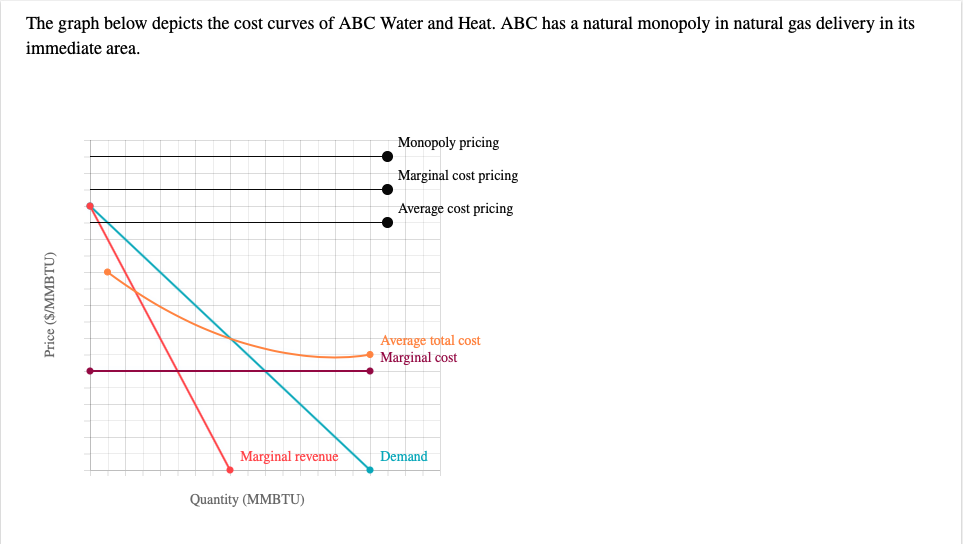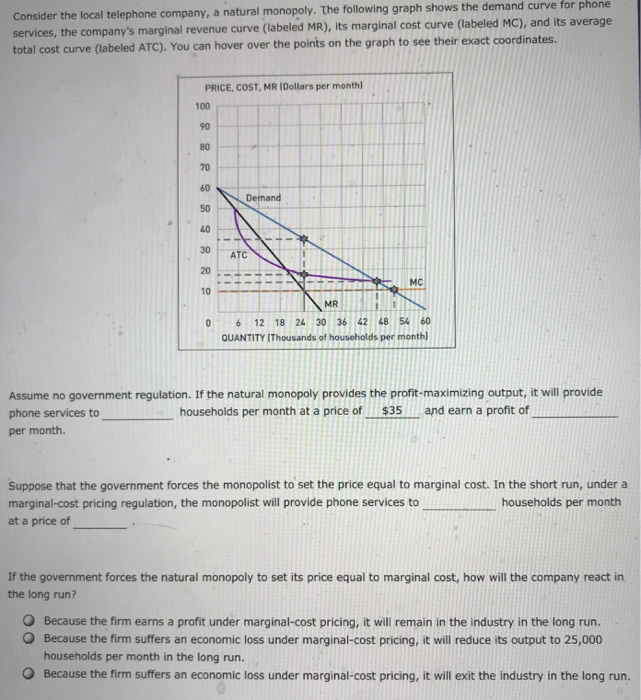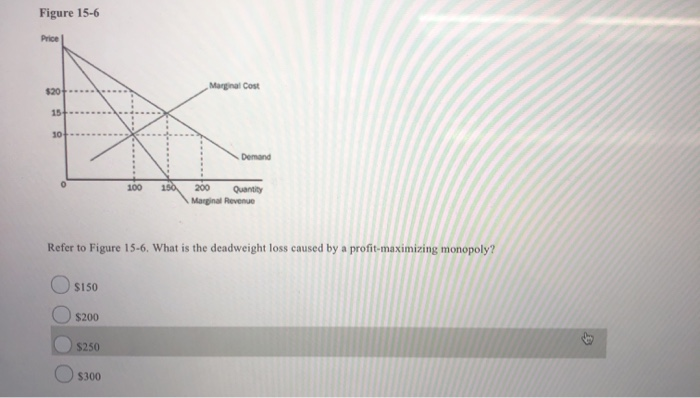Question

# 1. Marginal cost pricing means that a firm charges Group of answer choices A price that...

1.

Marginal cost pricing means that a firm charges

A price that is marginally lower than the average total cost of production.

Any price as long as average total cost is greater than marginal cost.

A price that is marginally higher than the average total cost of production

A price that is equal to the marginal cost of production.

2.

If the government wants a natural monopolist to achieve allocative efficiency, the government should

Ensure that the firm produces at full capacity.

Use price ceilings so the firm will earn a normal profit.

Regulate the firm so that it produces the output level at which economic profit is zero.

Subsidize the firm and require marginal cost pricing.

3.

An unregulated natural monopoly can lead to

Marginal cost pricing.

Loss of economies of scale.

Higher prices for consumers.

An optimal mix of output.

4.

If a natural monopoly is forced to set a price consistent with price efficiency, it will

Earn a profit on every unit of output produced.

Set price above marginal cost.

Incur a loss on every unit of output produced.

Set price equal to the ATC of production.

5.

The best way to address a natural monopoly without dismantling the economies of scale is

Laissez faire.

Antitrust.

Deregulation.

Regulation.

1) Solution: price that is equal to the marginal cost of production

Explanation: Marginal cost pricing means that the producer would produces up to the output where for a given market price equals the marginal cost of production

2) Solution: Subsidize the firm and require marginal cost pricing

Explanation: to achieve allocative efficiency for a natural monopolist the government would subsidize the firm and need marginal cost pricing

3) Solution: Higher prices for consumers

Explanation: The unregulated natural monopoly would charge consumer a high price

4) Solution: Incur a loss on every unit of output produced

Explanation: To set a consistent price with price efficiency in natural monopoly the producer incur a loss on every unit of produced output

5) Solution: Regulation

Explanation: The best approach for a natural monopoly without dismantling the economies of scale would be a proper regulation

#### Earn Coins

Coins can be redeemed for fabulous gifts.

Similar Homework Help Questions
• ### 7) If the government forces a monopoly to set its price equal to its marginal cost...

7) If the government forces a monopoly to set its price equal to its marginal cost and there are high economies of scale: Group of answer choices the monopolist’s costs will exceed revenue. the market will be more efficient than if the prices was equal to average cost. the monopolist is more likely to innovate. the monopolist will earn more profit than if they were unregulated. 8) If production costs and profits are similar, in a competitive market prices will...

• ### 1)In the U.S. a drug company’s patent remains in force for: Group of answer choices a)10...

1)In the U.S. a drug company’s patent remains in force for: Group of answer choices a)10 years. b)25 years. c)70 years. d)20 years. 2)When a natural monopoly exists in a given industry, the per-unit costs of production will be Group of answer choices a)lower for the smaller firms than for larger firms. b)lowest when there are a large number of producers in the industry. c)lowest when a single firm generates the entire output of the industry. d)minimized at the output...

• ### A natural monopoly is most likely to result if a single firm: Group of answer choices...

A natural monopoly is most likely to result if a single firm: Group of answer choices is the only seller in a community. is investor-owned, but is granted the exclusive right by the government to operate in a market. experiences economies of scale over a wide range of output. has gained control over a strategic input of an important production process.

• ### 1) The "Profit-Max/Loss-Min/Shutdown Rule" applies to: Group of answer choices Pure Monopoly only Perfect Competition only...

1) The "Profit-Max/Loss-Min/Shutdown Rule" applies to: Group of answer choices Pure Monopoly only Perfect Competition only Most market structures All market structures 3) A firm in a monopoly market structure always operates at an economic profit. Group of answer choices True False 4) Comparing monopoly and competitive market structures, "Deadweight Loss" refers to: Group of answer choices Underground markets developing to supply the monopoly good. Shortages caused by high monopoly pricing. The production gap resulting from under-allocation of resources. Surpluses...

• ### 1A Marginal revenue for a monopoly firm is: not related to the price that the monopolist...

1A Marginal revenue for a monopoly firm is: not related to the price that the monopolist charges for its products. less than the price that the monopolist charges for its products. always greater than the price that the monopolist charges for its products. equal to the price that the monopolist charges for its products. 1B Regarding monopoly firms, our text concludes that: firms which have been granted monopoly status by a government are less-efficient and provide a lower-quality and higher-priced...

• ### The graph below depicts the cost curves of ABC Water and Heat. ABC has a natural...The graph below depicts the cost curves of ABC Water and Heat. ABC has a natural monopoly in natural gas delivery in its immediate area. Monopoly pricing Marginal cost pricing Average cost pricing Price (\$/MMBTU) Average total cost Marginal cost Marginal revenue Demand Quantity (MMBTU) a. Place the point labelled “Monopoly pricing" at the appropriate coordinates to indicate the monopoly price and quantity b. Suppose the government tries to achieve allocative efficiency (P = MC) by imposing a marginal cost...

• ### Sketch a natural monopoly firm under marginal cost pricing regulation. Label its price, quantity, and profit....

Sketch a natural monopoly firm under marginal cost pricing regulation. Label its price, quantity, and profit. What is the deadweight loss (loss in consumer and producer surplus) if regulation is effective?

• ### Consider the local telephone company, a natural monopoly. The following graph shows the demand curve for...Consider the local telephone company, a natural monopoly. The following graph shows the demand curve for phone services, the company's marginal revenue curve (labeled MR), its marginal cost curve (labeled MC), and its average total cost curve (labeled ATC). You can hover over the points on the graph to see their exact coordinates. PRICE, COST, MR (Dollars per month) 100 90 80 70 60 Demand 50 40 30 ATC 20 MC 10 MR 54 60 30 36 42 48 0...

• ### please answer all questions! Figure 15-6 Price \$20+ Marginal Cost 100 150 200 Quantity Marginal Revenue...please answer all questions! Figure 15-6 Price \$20+ Marginal Cost 100 150 200 Quantity Marginal Revenue Refer to Figure 15-6. What is the deadweight loss caused by a profit-maximizing monopoly? O O \$150 \$200 \$250 Os300 A monopolist faces market demand given by P - 60 - Q. For this market, MR = 90 - 2Q and MC - Q. What price will the monopolist charge in order to maximize profits? O \$20 O \$30 O so Osso In Canada,...

• ### Under the cartel model, each firm produces where Group of answer choices marginal cost equals marginal...

Under the cartel model, each firm produces where Group of answer choices marginal cost equals marginal revenue. price equals marginal cost. the average cost curve is at a minimum. price exceeds marginal cost by the greatest amount.